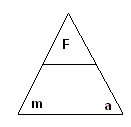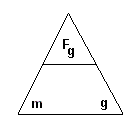#### Forces - Equation Triangles

You can use the following triangles to remember your formulas

• Force - Newton's Second Law• Force of Gravity - g = 9.8 m/s2• Friction Force - µ = coefficient of Friction - FN = m X g